SYLLABUS  Previous: 1.3.1 Advection  Up: 1.3 Prototype problems  Next: 1.3.3 Dispersion

1.3.2 Diffusion

Slide : [ diffusion - Green function || VIDEO login]

At the microscopic scale, diffusion is in fact related to a random motion leading to the prototype equation (exercise 1.04)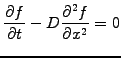(1.3.2#eq.1)

where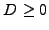is the diffusion coefficient. For a homogeneous medium, the combined advection-diffusion equation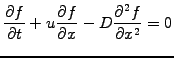(1.3.2#eq.2)

can be solved analytically in terms of the Green function (exercise 1.03)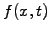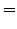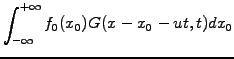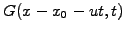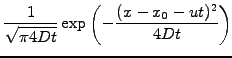(1.3.2#eq.3)

A numerical solution is generally required to solve the equation in an inhomogeneous medium, where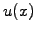,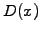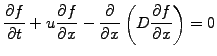Below is an example of a numerical solution describing the diffusion of an initial box function computed using the finite element method from chapter 3.

 Numerical experiments: diffusion Switch from an initial Box to a Cosine function and vary the ICWavelength parameter in powers of two: 2, 4, 8, 16, 32. Observe how short spatial scales decay more rapidly than larger scales: measure the decay rate as a function of the wavelength and try to reproduce the analytical result below. Back to the the initial Box function, observe how sharp edges disappear quickly, while large scale structures gradually evolve into Gaussians - reminder of the Green function in the analytical solution. Add a finite advection Velocity=0.2 and observe what happens when advection and diffusion are combined.

A harmonic ansatz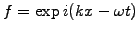can be used to analyze the diffusion equation and leads to the dispersion relation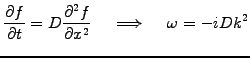(1.3.2#eq.4)

This shows that short wavelengths (large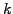) are more strongly damped (large negative imaginary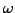) than long wavelengths, with a decay rate proportional to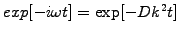. This explains the numerical experiments above.

Note that the heat equation, which describes the evolution of the temperature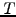in a medium with a heat conductivity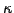in the presence of heat sources and sinks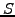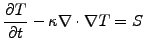(1.3.2#eq.5)

is one particular application of the diffusion equation. It can easily be reduced to (1.3.2#eq.1) by substituting the differential operator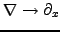in cartesian coordinates for a 1D slab.

SYLLABUS  Previous: 1.3.1 Advection  Up: 1.3 Prototype problems  Next: 1.3.3 Dispersion